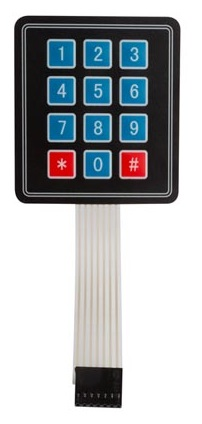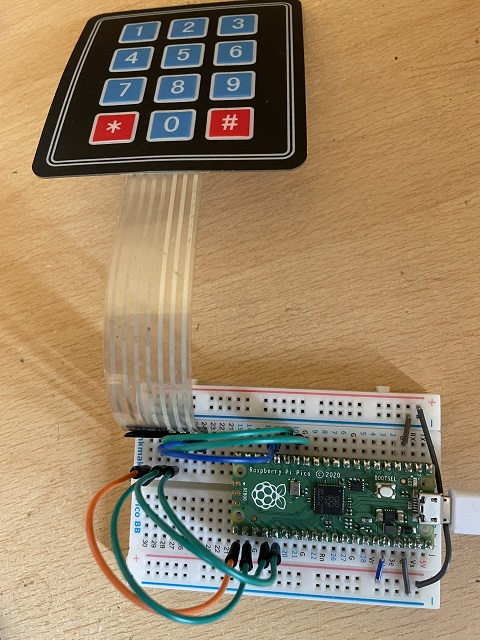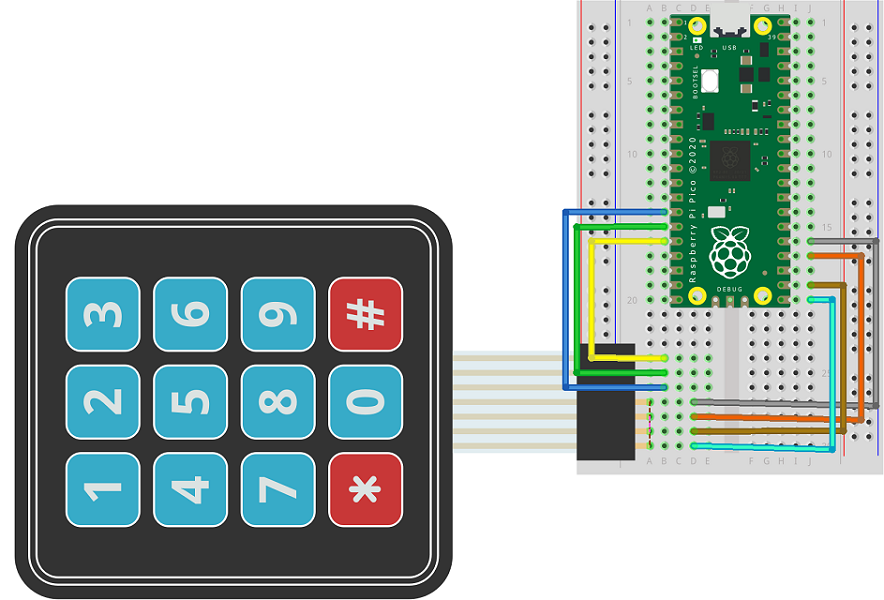# Raspberry Pi Pico 3x4 Membrane Matrix Keypad

There are several variants of the keypad I am using here. The one I have is a 3x4 number pad labelled with digits 0-9, * and #.These keypads are relatively cheap. You can get this for under £4 from Pimoroni.

The keypad is a matrix in the sense that the switches are connected on one pin along each of the three columns and on the other pin on each of the four rows. Looking closely at the end of the ribbon cable, you can see that the first four pins (left in the image) are different from the other three.Here is the Fritzing diagram for this circuit,The columns are set to be inputs and use an internal pull up resistor as you would for a pushbutton. The rows are outputs and are all set to high. This prevents the columns from being grounded. To read the keypad, each row is set to low in turn. Then all of the columns are read to see if one is also low (ie switch pressed). If not, the row is set to high and the next one checked.

```from machine import Pin
from time import sleep

cols = [Pin(i, Pin.IN, Pin.PULL_UP) for i in [10,11,12]]
rows = [Pin(i, Pin.OUT) for i in [16,17,18,19]]

for r in rows:
r.value(1)

["4","5","6"],
["7","8","9"],
["*","0","#"]]

for r in rows:
r.value(0)
reading = [c.value() for c in cols]
r.value(1)
return this_key
r.value(1)
return "Z"

while True: